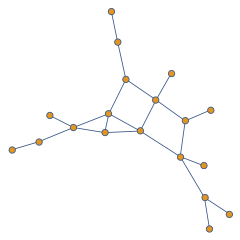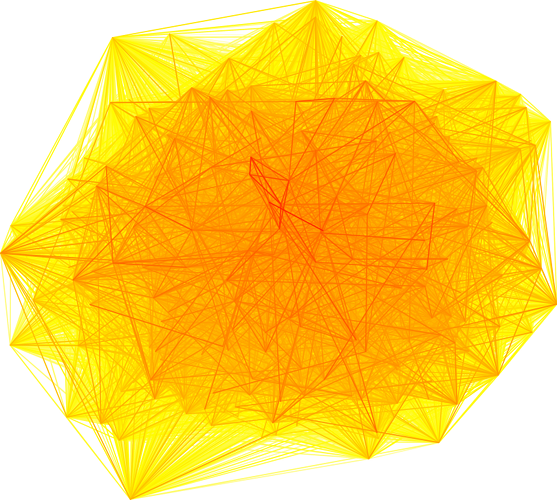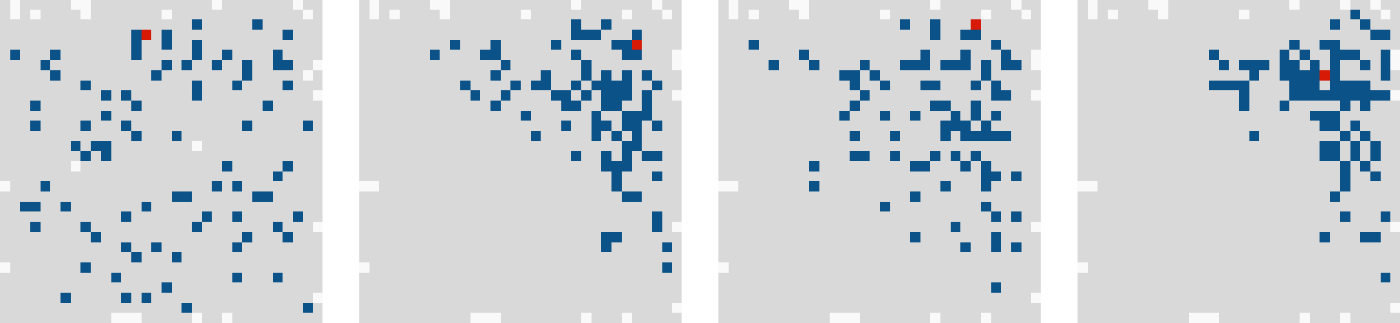## Graph layout on a constrained grid

Posted on 1st August 2018
by Rupert Overall

A network (or in mathematical terminology, a graph) is a collection of entities (known as nodes or vertices) connected by links (also known as edges). Many types of data can be very usefully represented by a network, which allows ease of visualisation and access to analytic tools from the field of graph theory. This post is concerned with visualisation. Specifically, the problem of how best to show the connectivity of vertices in a large graph. To better explain the problem, a little more background might be needed.

### Gene correlation networks

One use of graphs/networks is to show how genes are related to each other. The nature of the connections between genes can be varied, but it is common to depict two genes as connected if they share similar transcript expression patterns. This is what the example data here will be based on. Thus, the vertices of the network are genes, and the edges are the correlation between the transcript expression pattern of the two connected genes. If the correlation is very strong, the two genes should be drawn close together in the ideal graph; genes less correlated are further apart. Using this simple rule, a graph can be drawn like this.###### A simple graph with vertices (the circles) connected by edges (the lines).

When applied to the c. 20000 transcripts in the whole genome, however, the network looks more like this.### Network percolation

When a particular gene of interest is studied, it is interesting to know which other genes it is similar to. This can be done by identifying its ‘neighbours’ in the network; i.e. those genes which are correlated/connected above some threshold value. The influence of the gene of interest can be further traced by looking at the 2nd-level neighbours (in other words, friends-of-friends). This concept can continue to 3rd-level, 4th-level and so on until all of the (reachable) vertices in the network have been accounted for. I note that this covers the reachable extent of the network; some vertices are not connected to each other by any path through any edges and thus exist in disjoint subnetworks. Obviously a signal can never percolate from one unconnected subnetwork to another.

Now, some genes are highly connected and have very many neighbours, and those neighbours might also be highly connected. This is important information because it implies that the effect of such a gene can percolate rapidly and strongly throughout the network. Such a gene is likely to be influential in that network. It would be interesting to visualise how well connected a gene is; in other words, how many neighbours it takes to cover the entire reachable network. This could be achieved by colouring the 1st neighbours a bold colour and the vertices that are connected by more neighbour steps in different colours. The task then would be to lay out the graph so that only the coloured vertices are visible (the edges are not informative in this view) and so that the distance between vertices is proportional to the strength of their correlation. This is where the GridGraph code comes in.

### The GridGraph algorithm

Because there is no inherent 2-dimensional shape of such a network (the network is actually n-dimensional, where n is the number of genes), I have chosen to draw the graph on a square canvas. I also do not want vertices to overlap, so I decide to draw them on a grid where each vertex occupies one grid cell. The problem, then, is to arrange the vertices on the grid so that the distance between each vertex is proprtional to the correlation strength. My solution to this problem was to initially lay out the vertices on the grid randomly and iteratively shuffle them to optimise inter-vertex distances. In fact, the approach I settled on was to identify the most highly-correlated neighbours and find their positions on the grid. Then the average x and y coordinates of these genes (the centroid of the 1st-level, or 1st-degree, cluster) was calculated; this is the optimal position of the gene of interest. A path between the centroid and the current gene position is calculated (this is a wonky “Manhattan” path of cells nearest the straight line connecting the two cells) and the target cell is taken to be the end point of a subset of this path so that the gene of interest moves closer, but not to the centroid. This allows the system to converge on a layout of genes that is a compromise between the ideal positions of each gene (an ideal solution simply does not exists when trying to squash >20000 dimensions into the 2 dimensions of the graph layout). Once the starting point of the path (the current cell that the gene of interest occupies) and the end point of the path (the cell that is part way (50% by default) toward the centroid) have been determined, the genes are shuffled in the following way; starting at the start, the next gene on the path moves to the start, the gene at path position 2 moves to position 1, the gene at path position 3 moves to position 2, and so on until the end of the path which is now an empty cell. The gene that was initially at the start (the gene of interest) moves to this end cell to complete the shuffle. This process is repeated for all genes. Then the whole ordeal is repeated again for a specified number of times (iterations).

### The GridGraph package

I have created an R package, GridGraph, which contains one (so far) layout function layout.grid.centroid.attraction that implements the algorithm described above. The function takes several parameters. Firstly distance.matrix is the required correlation network as a distance matrix. Then a threshold is required, which defines which other vertices are used to calculate the centroid; all vertices which have a distance below the threshold will be used for this. The optional scaling factors row.size.factor and col.size.factor control how big the grid is on which the layout is performed. By default, this is a square grid with the minimum number of cells such that the whole distance matrix fits comfortably. It can be nice to add a bit of a buffer of empty cells so that the actual graph is more free to converge sensibly. The algorithm used means that the empty cells will tend to be pushed to the periphery (in fact, they are in all the runs I have performed so far) so this results in a (default white) border around the graph. A further option increment defines how far along the start-centroid path the vertex will be moved when shuffling. The default is 0.5 (i.e. halfway along the path). If this is set lower, the layout will converge more slowly. If it is set too high, then the path shuffling will tend to stomp all over the updated positions of previous vertices and it is likely that the algorithm will not converge to a stable layout. A helpful option is extend which skips the initial randomisation of the layout and allows additional iterations to be performed (rather than having to redo the iterations already performed so far). Obviously the other parameters should be kept the same if this option is to be used otherwise interpretation of the results will be complicated. Importantly, the random seed should be the same if you want the layout to be reproducible. By default in R, random numbers are generated using a seed created from the current time; but you can set this using set.seed. Finally, the option iterations specifies how many times the algorithm cycles through all of the vertices. The default setting is 1. I have found that, even for a whole-transcriptome dataset with around 20000 vertices, the layout seems to converge in under 20 iterations, but this is something that needs to be empirically determined in each case. The options extend and iterations used together allow a snapshot of the layout to be saved at regular intervals during convergence. This is kind of fun when the images are stitched together to make a movie. The randomly-distributed vertices jiggle around and swarm to a mutually optimal arrangement as the algorithm runs. As well as looking quite cool, this is helpful to see when the layout converges. A few extra commands and tools are needed to create the movie – I will show one method below.

### An example

To install the package, use install.packages with the latest version of the package from my software page (at the time of writing, I just released version 1.0).

``install.packages('http://research.rupertoverall.net/resources/gridgraph/GridGraph_1.0.1.tar.gz', repos = NULL)``

And then load the package into the workspace;

``require(GridGraph)``

There is an example dataset (expression data for 1000 genes) included in the package. We now load this and create a distance matrix from it (just the Pearson correlation matrix converted into distances, so that vertices/genes with high positive correlations are drawn closer to each other).

``````data(hipExpr)
network = cor(hipExpr, method="pearson", use="pairwise.complete.obs")
distance.matrix = 1 - (network * (network >= 0))``````

Next we can find all the genes that are close (below a threshold distance) to a gene of interest (a ‘seed’ gene). We would expect these vertices to cluster together, which we should be able to observe as the layout algorithm does it’s stuff.

``````threshold = 0.5
# Colours by vertex neigbourhood
seed.vertex = match("ENSMUSG00000004891", rownames(distance.matrix))
seed.neighbours = which(distance.matrix[seed.vertex, ] <= threshold)``````

We can now actually run the layout. This initial iteration will be the starting point for the iterations performed in the loop below.

``````# Set the seed for the random number generator
set.seed(1)
# The variable 'grid.layout' will be updated at each iteration in the loop and will be used as the starting point for the next iteration
grid.layout = layout.grid.centroid.attraction(distance.matrix, threshold, iterations = 1)``````

To observe the behaviour of our seed gene and its friends, we need to give these vertices different colours. I have set the background colour to be #FAFAFA (the background colour of this page). This is used to plot the NA values which are just there to pad out the distance.matrix data so that they fit in a square matrix. All vertices are a pale grey (#E0E0E0). Then the vertices of interest are re-coloured; firstly the neighbours are set to blue (#006699) and finally the seed vertex is coloured red (#E03000). Now we can see how these vertices are plotted in relationship to each other. When we plot the layout from the initial iteration using these colours, we can see that the vertices are scattered all over the place. This is because we just laid them out randomly (although the random number generator has been given a seed, so you will get the same pattern as shown here if you run this code again).

``````# Set up the colour matrix
grid.plot.colours = matrix(rep("#E0E0E0", length(grid.layout)), ncol=ncol(grid.layout))
grid.plot.colours[is.na(grid.layout)] = "#FAFAFA"
grid.plot.colours[match(seed.neighbours, grid.layout)] = "#006699"
grid.plot.colours[match(seed.vertex, grid.layout)] = "#E03000"

# Plot first iteration just to test
grid.plot(grid.layout, grid.plot.colours)``````

Now we can have some fun running some iterations and plotting each iteration as we go. The algorithm will converge on some optimal layout and we can see how it does this. Each iteration will generate a PNG image. I have put these into a subdirectory (‘Movie’) to avoid clutter. We can join these into an animation when we’re done.

``````# Create a subdirectory 'Movie' in the working directory to hold the individual images
dir.create("Movie")``````

I now run 50 iterations, giving each PNG image a sequential name. Note that the layout function layout.grid.centroid.attraction has been given the previous existing layout as a parameter extend so that our iterations build on the converging layout step-by-step.

``````for(iteration in 1:50){
# Run just one iteration at a time to see the convergence
# Extend = the 'grid.layout' of the previous iteration, which is used to set the initial state
grid.layout = layout.grid.centroid.attraction(distance.matrix, threshold, iterations = 1, extend = grid.layout)
# Set up the colour matrix
grid.plot.colours = matrix(rep("#E0E0E0", length(grid.layout)), ncol=ncol(grid.layout))
grid.plot.colours[is.na(grid.layout)] = "#FFFFFF"
grid.plot.colours[match(seed.neighbours, grid.layout)] = "#006699"
grid.plot.colours[match(seed.vertex, grid.layout)] = "#E03000"
# All the PNG image files will be saved in the 'Movie' directory
png(file=sprintf("Movie/Image_%04d.png", iteration), width=85, height=85, units="mm", res=150)
grid.plot(grid.layout, grid.plot.colours)
dev.off()
}``````

The images below show four of these images demonstrating how the converging layout means that the correlated neighbours are drawn increasingly close together. Of course, every single vertex is trying to group into its own cluster, so there is competition between solutions that are ‘optimal’ for each vertex. The algorithm finds some solution that is a global compromise.###### Images of the layout at different stages of convergence. The correlated vertices tend to cluster as expected.

Now we can compile a movie. This step is very platform dependent, so you will need to experiment. I ran this on an Apple laptop running OS X. The Unix program ffmpeg can be installed on OS X using homebrew. The same code works on Linux. There are many other solutions including GUI tools (in fact there are some R options as well I believe – I will look into this if I get time).

``````# Movie
# Remove the movie file if it already exists (from a previous run)